I have several web pages intended for students; this seems to be the most popular one. FONTS FINALLY REPAIRED November 2009.

Browser adjustments: This web page uses subscripts, superscripts, and unicode symbols. The latter may display incorrectly on your computer if you are using an old browser and/or an old operating system.

# THE MOST COMMON ERRORS IN UNDERGRADUATE MATHEMATICS

This web page describes the errors that I have seen most frequently in undergraduate mathematics, the likely causes of those errors, and their remedies. I am tired of seeing these same old errors over and over again. (I would rather see new, original errors!) I caution my undergraduate students about these errors at the beginning of each semester.   Outline of this web page:

(There is some overlap among these topics, so I recommend reading the whole page.) ... Of related interest: Paul Cox's web page, and the books of Bradis, Minkovskii, and Kharcheva and E. A. Maxwell.

Ultimately, what are the sources of errors and of misunderstanding? What kinds of biases and erroneous preconceptions do we have? Two of my favorite historic discoveries are Einstein's discovery of relativity and Cantor's discoveries of some of the most basic rules of infinities. These discoveries are remarkable in that neither involved long, involved, complicated computations. Both are fairly simple, in retrospect, to anyone who has studied them. But both involved "thinking outside the box" par excellence -- i.e., seeing past the assumptions that were inherent in our culture and our language. As philosopher John Culkin said, "We don't know who discovered water, but we are certain it wasn't a fish." That certain mathematical errors are common among students may be partly a consequence of biases that are built into our language and culture, some of which we aren't even aware of.

## Errors in Communication

Some teachers are hostile to questions. That is an error made by teachers. Teachers, you will be more comfortable in your job if you try to do it well, and don't think of your students as the enemy. This means listening to your students and encouraging their questions. A teacher who only lectures, and does not encourage questions, might as well be replaced by a book or a movie. To teach effectively, you have to know when your students have understood something and when they haven't; the most efficient way to discover that is to listen to them and to watch their faces. Perhaps you identify with your brightest students, because they are most able to appreciate the beauty of the ideas you are teaching -- but the other students have greater need of your help, and they have a right to it.

A variant of teacher hostility is teacher arrogance. In its mildest form, this may simply mean a teacher who, despite being polite and pleasant, is unable to conceive of the idea that he/she could have made an error, even when that error is brought directly to his/her attention. Actually, most of the errors listed below can be made by teachers, not just by students. (However, most teachers are right far more often than their students, so students should exercise great caution when considering whether their teachers could be in error.)

If you're a student with a hostile teacher, then I'm afraid I don't know what advice to give you; transfer to a different section or drop the course altogether if that is feasible. The remarks on communication in the next few paragraphs are for students whose teachers are receptive to questions. For such students, a common error is that of not asking questions.

If you think your teacher may have made a mistake on the chalkboard, you'd be doing the whole class a favor by asking about it. (To save face, just in case the error is your own, formulate it as a question rather than a statement. For instance, instead of saying "that 5 should be a 7", you can ask "should that 5 be a 7?")

And try to ask your question as soon as possible after it comes up. Don't wait until the very end of the example, or until the end of class. As a teacher, I hate it when class has ended and students are leaving the room and some student comes up to me and says "shouldn't that 5 have been a 7?" Then I say "Yes, you're right, but I wish you had asked about it out sooner. Now all your classmates have an error in the notes that they took in class, and they may have trouble deciphering their notes later."

In the early 1980s, I managed a computer retail store. Several of my employees were college students. One bright your man was having difficulty with his Freshman college algebra class. I tutored him and he did very well, but invariably, he would say, "the professor worked through this problem on the board, and it was nothing like this. I sure hope we got the correct answer."

I accompanied him to class one morning and discovered the source of his frustration. The professor was from the music department, and didn't normally teach college algebra --- he had been pressed into duty when over enrollment forced the class to be split.

During the class, he picked a problem from the assignment to work out on the board. Very early in the problem, he made an error. I don't recall the specifics, but I'm sure it was one of the many typical algebra errors you list.

Because of the error, he eventually reached a point from which he could no longer proceed. Rather than admitting an error and going to work to find it, he paused staring at the board for several seconds, then turned to the class and said, "...and the rest, young people, should be obvious."

Unclear wording. The English language was not designed for mathematical clarity. Indeed, most of the English language was not really designed at all -- it simply grew. It is not always perfectly clear. Mathematicians must build their communication on top of English [or replace English with whatever is your native or local language], and so they must work to overcome the weaknesses of English. Communicating clearly is an art that takes great practice, and that can never be entirely perfected.

Lack of clarity often comes in the form of ambiguity -- i.e., when a communication has more than one possible interpretation. Miscommunication can occur in several ways; here are two of them:

• One of the things that you've said has two or more possible meanings, and you're aware of that fact, but you're satisfied that it's clear which meaning you intended -- either because it's clear from the context, or because you've added some further, clarifying words. But your audience isn't as knowledgeable as you about this subject, and so the distinction was not clear to them from the context or from your further clarifying words. Or,
• One of the things that you've said has two or more possible meanings, and you're not aware of that fact, because you weren't watching your own choice of words carefully enough and/or because you're not knowledgeable enough about some of the other meanings that those words have to some people.

One way that ambiguity can occur is when there are multiple conventions. A convention is an agreed-upon way of doing things. In some cases, one group of mathematicians has agreed upon one way of doing things, and another group of mathematicians has agreed upon another way, and the two groups are unaware of each other. The student who gets a teacher from one group and later gets another teacher from the other group is sure to end up confused. An example of this is given under "ambiguously written fractions," discussed later on this page.

Choosing precise wording is a fine art, which can be improved with practice but never perfected. Each topic within math (or within any field) has its own tricky phrases; familiarity with that topic leads to eventually mastering those phrases.

For instance, one student sent me this example from combinatorics, a topic that requires somewhat awkward English:

How many different words of five letters can be formed from seven different consonants and four different vowels if no two consonants and vowels can come together and no repetitions are allowed? How many can be formed if each letter could be repeated any number of times?
There are a number of places where this problem is unclear. In the first sentence, I'm not sure what "can come together" means, but I would guess that the intended meaning is
How many different words of five letters can be formed from seven different consonants and four different vowels if no two consonants can occur consecutively and no two vowels can occur consecutively and no repetitions are allowed?
The second sentence is a bit worse. The student misinterpreted that sentence to mean
How many different words of five letters can be formed from seven different consonants and four different vowels if each letter could be repeated any number of times?
But usually, when a math book asks two consecutive questions related in this fashion, the second question is intended as a modification of the first question. We are to retain all parts of the first question that are compatible with the new conditions, and to discard all parts of the first question that would be contradicted by the new conditions. Thus, the second sentence in our example should be interpreted in this rather different fashion, which yields a different answer:
How many different words of five letters can be formed from seven different consonants and four different vowels if no two consonants can be consecutive, no two vowels can be consecutive, and each letter could be repeated any number of times?

Bad handwriting is an error that the student makes in communicating with himself or herself. If you write badly, your teacher will have difficulty reading your work, and you may even have difficulty reading your own work after some time has passed!

Usually I do not deduct points for a sloppy handwriting style, provided that the student ends up with the right answer at the end -- but some students write so badly that they end up with the wrong answer because they have misread their own work. For instance,

∫ (5x4+2)dx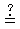x5+7x+C (should be x5+2x+C)
This student's handwriting was so bad that he misread his own writing; he took the "2" for a "7". You'll have to use your imagination here, since this electronic typesetting cannot duplicate sloppy handwriting. You do not need to make your handwriting as neat as this typeset document, but you need to be neat enough so that you or anyone else can distinguish easily between characters that are intended to be different. Most students would fare better if they would print their mathematics, instead of using cursive writing.

By the way, write your plus sign (+) and lower-case letter Tee (t) so that they don't look identical! One easy way to do this is to put a little "tail" at the bottom of the t, just as it appears in this typeset document. (I assume that the fonts you're using on your browser aren't much different from my fonts.)

Not reading directions. Students often do not read the instructions on a test carefully, and so in some cases they give the right answer to the wrong problem.

Loss of invisible parentheses. This is not an erroneous belief; rather, it is a sloppy technique of writing. During one of your computations, if you think a pair of parentheses but neglect to write them (for lack of time, or from sheer laziness), and then in the next step of your computation you forget that you omitted a parenthesis from the previous step, you may base your subsequent computations on the incorrectly written expression. Here is a typical computation of this sort:

3 ∫ (5x4+7)dx3x5+7x+C
But that should be
3 ∫ (5x4+7)dx = 3(x5+7x)+C = 3x5+21x+C
That's an entirely different answer, and it's the correct answer. To see where the error creeps in, just try erasing the last pair of parentheses in the line above.

A partial loss of parentheses results in unbalanced parentheses. For example, the expression "3(5x4+2x+7" is meaningless, because there are more left parentheses than right parentheses. Moreover, it is ambiguous -- if we try to add a right parenthesis, we could get either "3(5x4+2x)+7" or "3(5x4+2x+7)"; those are two different answers.

Loss of parentheses is particularly common with minus signs and/or with integrals; for instance,

–∫ (5x4–7)dx–x5–7x+C (should be –x5+7x+C)

Terms lost inside an ellipsis. An ellipsis is three dots (...), used to denote "continue the pattern". This notation can be used to write a long list. For instance, "1, 2, 3, ..., 100" represents all the integers from 1 to 100; that's much more convenient than actually writing all 100 numbers. And for some purposes, an ellipsis is not just a convenience, it's a necessity. For instance, "1, 2, 3, ..., n" represents all the integers from 1 to n, where n is some unspecified positive integer; there's no way to write that without an ellipsis.

The ellipsis notation conceals some terms in the sequence. But can only be used if enough terms are left unconcealed to make the pattern evident. For instance, "1, ..., 64" is ambiguous -- it might have any of these interpretations:

• "1, 2, 3, 4, ..., 64"     (all the integers from 1 to 64)
• "1, 4, 9, 16, 25, 36, 49, 64"     (that's n2 as n goes from 1 to 8)
• "1, 2, 4, 8, 16, 32, 64"     (that's 2n as n goes from 0 to 6)
Of course, in some cases one of these meanings might be clear from the context. And just how much information is needed "to make a pattern evident" is a subjective matter; it may vary from one audience to another. Best to err on the safe side: give at least as much information as would be needed by the least imaginative member of your audience.

I have seen many errors in using ellipses when I've tried to teach induction proofs. For instance, suppose that we'd like to prove

[*n]       12 + 22 + 32 + ... + n2   =   n(n+1)(2n+1)/6
for all positive integers n. The procedure is this: Verify that the equation is true when n=1 (that's the "initial step); then assume that [*n] is true for some unspecified value of n and use that fact to prove that it's true for the next value of n -- i.e., to prove [*(n+1)] (that's the "transition step"). Here is a typical error in the transition step: Add 2n+1 to both sides of [*n]. Thus we obtain
[i]     12 + 22 + 32 + ... + n2 + 2n+1   =   (2n+1) + n(n+1)(2n+1)/6.
But that says
[ii]     12 + 22 + 32 + ... + (n+1)2   =   (2n+1) + n(n+1)(2n+1)/6.
We've made a mistake already, in the left side of the equation. (Can you find it? I'll explain it in a moment.) Now make some algebra error while rearranging the right side of the equation, to obtain
[*(n+1)]     12 + 22 + 32 + ... + (n+1)2   =   (n+1)(n+2)(2n+3)/6.
And now it appears that we're done. But there was an algebra error on the right side: (2n+1) + n(n+1)(2n+1)/6 actually is not equal to (n+1)(n+2)(2n+3)/6. (You can check that easily.)

The error on the left side was more subtle. It is based on the fact that too many terms were concealed in the ellipsis, and so the pattern was not revealed accurately. To see what is really going on, let's rewrite equations [i] and [ii], putting more terms in:

[i]     12 + 22 + 32 + ... + (n-2)2 + (n-1)2 + n2 + 2n+1   =   (2n+1) + n(n+1)(2n+1)/6.

[ii]     12 + 22 + 32 + ... + (n-2)2 + (n-1)2 + (n+1)2   =   (2n+1) + n(n+1)(2n+1)/6.

And now you can see that the left side is missing its n2 term, so the left side of [ii] is not equal to the left side of [*(n+1)].

## Algebra Errors

Sign errors are surely the most common errors of all. I generally deduct only one point for these errors, not because they are unimportant, but because deducting more would involve swimming against a tide that is just too strong for me. The great number of sign errors suggests that students are careless and unconcerned -- that students think sign errors do not matter. But sign errors certainly do matter, a great deal. Your trains will not run, your rockets will not fly, your bridges will fall down, if they are constructed with calculations that have sign errors.

Sign errors are just the symptom; there can be several different underlying causes. One cause is the "loss of invisible parentheses," discussed in a later section of this web page. Another cause is the belief that a minus sign means a negative number. I think that most students who harbor this belief do so only on an unconscious level; they would give it up if it were brought to their attention. [My thanks to Jon Jacobsen for identifying this error.]

Is –x a negative number? That depends on what x is.

• Yes, if x is a positive number.
• No, if x itself is a negative number. For instance, when x = –6, then –x = 6 (or, for emphasis, –x = +6).
That's something like a "double negative". We sometimes need double negatives in math, but they are unfamiliar to students because we generally try to avoid them in English; they are conceptually complicated. For instance, instead of saying "I do not have a lack of funds" (two negatives), it is simpler to say "I have sufficient funds" (one positive).

Another reason that some students get confused on this point is that we read "–x" aloud as "minus x" or as "negative x". The latter reading suggests to some students that the answer should be a negative number, but that's not right. [Suggested by Chris Phillips.]

Misunderstanding this point also causes some students to have difficulty understanding the definition of the absolute value function. Geometrically, we think of |x| as the distance between x and 0. Thus |–3| = 3 and |27.3| = 27.3, etc. A distance is always a positive quantity (or more precisely, a nonnegative quantity, since it could be zero). Informally and imprecisely, we might say that the absolute value function is the "make it positive" function.

Those definitions of absolute value are all geometric or verbal or algorithmic. It is useful to also have a formula that defines |x|, but to do that we must make use of the double negative, discussed a few sentences ago. Thus we obtain this formula: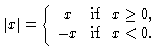which is a bit complicated and confuses many beginners. Perhaps it's better to start with the distance concept.

Many college students don't know how to add fractions. They don't know how to add (x/y)+(u/v), and some of them don't even know how to add (2/3)+(7/9). It is hard to classify the different kinds of mistakes they make, but in many cases their mistakes are related to this one:

Everything is additive. In advanced mathematics, a function or operation f is called additive if it satisfies f(x+y)=f(x)+f(y) for all numbers x and y. This is true for certain familiar operations -- for instance,

• the limit of a sum is the sum of the limits,
• the derivative of a sum is the sum of the derivatives,
• the integral of a sum is the sum of the integrals.
But it is not true for certain other kinds of operations. Nevertheless, students often apply this addition rule indiscriminately. For instance, contrary to the belief of many students,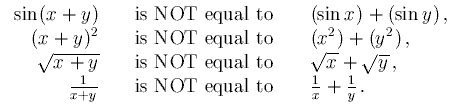We do get equality holding for a few unusual and coincidental choices of x and y, but we have inequality for most choices of x and y. (For instance, all four of those lines are inequalities when x = y = π/2. The student who is not sure about all this should work out that example in detail; he or she will see that that example is typical.)

One explanation for the error with sines is that some students, seeing the parentheses, feel that the sine operator is a multiplication operator -- i.e., just as 6(x+y)=6x+6y is correct, they think that sin(x+y)=sin(x)+sin(y) is correct.

The "everything is additive" error is actually the most common occurrence of a more general class of errors:

Everything is commutative. In higher mathematics, we say that two operations commute if we can perform them in either order and get the same result. We've already looked at some examples with addition; here are some examples with other operations. Contrary to some students' beliefs,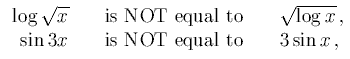etc. Another common error is to assume that multiplication commutes with differentiation or integration. But actually, in general (uv)′ does not equal (u′)(v′) and ∫ (uv) does not equal (∫ u)(∫ v).

However, to be completely honest about this, I must admit that there is one very special case where such a multiplication formula for integrals is correct. It is applicable only when the region of integration is a rectangle with sides parallel to the coordinate axes, and

u(x) is a function that depends only on x (not on y), and
v(y) is a function that depends only on y (not on x).
Under those conditions,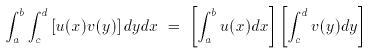(I hope that I am doing more good than harm by mentioning this formula, but I'm not sure that that is so. I am afraid that a few students will write down an abbreviated form of this formula without the accompanying restrictive conditions, and will end up believing that I told them to equate ∫ (uv) and (∫ u)(∫ v) in general. Please don't do that.)

Undistributed cancellations. Here is an error that I have seen fairly often, but I don't have a very clear idea why students make it.

 (3x+7)(2x–9) + (x2+1) (3x+7) (2x–9) + (x2+1) (2x–9) + (x2+1) f(x) == (3x+7)(x3+6) (3x+7) (x3+6) (x3+6)

In a sense, this is the reverse of the "loss of invisible parentheses" mentioned earlier; you might call this error "insertion of invisible parentheses." To see why, compare the preceding computation (which is wrong) with the following computation (which is correct).

 (3x+7) [ (2x–9) + (x2+1)] (3x+7) [ (2x–9) + (x2+1) ] (2x–9) + (x2+1) g(x) = = = (3x+7) (x3+6) (3x+7) (x3+6) (x3+6)

Apparently some students think that f(x) and g(x) are the same thing -- or perhaps they simply don't bother to look carefully enough at the top line of f(x), to discover that not everything in the top line of f(x) has a factor of (3x+7). If you still don't see what's going on, here is a correct computation involving that first function f :

 x2+1 2x–9 + (3x+7)(2x–9) + (x2+1) 3x+7 f(x) = = (3x+7)(x3+6) x3+6
Why would students make errors like these? Perhaps it is partly because they don't understand some of the basic concepts of fractions. Here are some things worth noting:
• Multiplication is commutative -- that is, xy = yx. Consequently, most rules about multiplication are symmetric. For instance, multiplication distributes over addition both on the left and on the right:
(x1+x2)y=(x1y)+(x2y)       and       x(y1+y2)=(xy1)+(xy2) .
• Division is not commutative -- in general, x/y is not equal to y/x. Consequently, rules about division are not symmetric (though perhaps some students expected them to be symmetric). For instance,
(x1+x2)/y = (x1/y)+(x2/y)       but in general       x/(y1+y2) ≠ (x/y1)+(x/y2) .
• Fractions represent division and grouping (i.e., parentheses). For instance, the fraction
 a+b c+d
is the same thing as (a+b)/(c+d). If you omit either pair of parentheses from that last expression, you get something entirely different. (Thanks to Mark Meckes for pointing out this possible explanation of the origin of such errors.) Perhaps some of the students' errors stem from such an omission of parentheses? or a lack of understanding of how important those parentheses are? That would seem to be indicated by the prevalance of another type of error described elsewhere on this page, "loss of invisible parentheses".

Dimensional errors. Most of this web page is devoted to things that you should not do, but dimensional analysis is something that you should do. Dimensional analysis doesn't tell you the right answer, but it does enable you to instantly recognize the wrongness of some kinds of wrong answers. Just keep careful track of your dimensions, and then see whether your answer looks right. Here are some examples:

• If the question is a word-problem, think about whether your answer makes sense. For instance, if you're given the dimensions of a coin and you're asked to find its surface area, and you come up with an answer of 3000 square miles, you should realize that you've probably made an error (even though your answer has the right units), and you should look for that error. (This is not really an example of dimensional analysis, but I didn't know where else to put it. Thanks to Sandeep Kanabar for this example.)
• Even if you don't remember the formula for the volume of a sphere of radius r, you know that it has to have a factor of r3, not r2 , so the answer couldn't possibly be πr2.
• Even if you don't remember the formula for the surface area of a sphere of radius r, you know it has to get small when r gets small. So it couldn't possibly be something like 2+3r2.

Here is a cute example of dimensional analysis (submitted by Benjamin Tilly).

Problem: Where has my money gone? My dollar seems to have turned into a penny:

$1 = 100¢ = (10¢)2 = ($0.10)2 = $0.01 = 1¢ Explanation: Of course, the problem is a disregard for dimensional units. Strictly speaking, if you square a dollar, you should get a square dollar. I don't know what a "square dollar" is, but I still know how to compute with it, and I know that a "square dollar" must be equal to 10,000 "square pennies", since one dollar is 100 pennies. Dimensional computations will not yield errors if we handle the dimensional units correctly. Here is a correct computation:$21 = ($1)2 = (100¢)2 = 1002¢2 = 10,000¢2. It should now be evident what was wrong with the first calculation: 100¢ is not equal to (10¢)2. It's true that the 100 is equal to the 102, but the ¢ is not equal to ¢2. Likewise, later in the computation,$2 is not equal to \$.

Idiosyncratic inverses. We need to be sympathetic about the student's difficulty in learning the language of mathematicians. That language is a bit more consistent than English, but it is not entirely consistent -- it too has its idiosyncrasies, which (like those of English) are largely due to historical accidents, and not really anyone's fault. Here is one such idiosyncrasy: The expressions sinn and tann get interpreted in different ways, depending on what n is.

sin2x = (sin x)2 and tan2x = (tan x)2;
but
sin–1x = arcsin(x) and tan–1x = arctan(x).
Some students get confused about this; some even end up setting arctan(x) equal to 1/(tan x). When I teach, I try to reduce confusion by always writing arcsin or arctan, rather than sin–1 or tan–1. But the sin–1 and tan–1 notation still needs to be discussed, as it is used on nearly all handheld calculators. Thanks to Ian Morrison and John Armerding for pointing this one out.

Confusion about the square root symbol. Every positive number b has two square roots. The expression √b actually means "the nonnegative square root of b," but unfortunately some students think that that expression means "either of the square roots of b" -- i.e., they think it represents two numbers. ... This error is made more common because of the unfortunate fact that we math teachers are merely human, and sometimes a little sloppy: When we write √b on the blackboard, what we say aloud might just be "the square root of b." But that's just laziness. If you ask us specifically about that, we'll tell you "Oh, I'm sorry, of course I meant the nonnegative square root of b; I thought that goes without saying." ... If you really do want to indicate both square roots of b, you use the plus-or-minus sign, as in this expression: ±√b.

Problems with order of operations. It is customary to perform certain mathematical operations in certain orders, and so we don't need quite so many parentheses. For instance, everyone agrees that "6w+5" means "(6w)+5", and not "6(w+5)" -- the multiplication is performed before the addition, and so the parentheses are not needed if "(6w)+5" is what you really mean to say. Unfortunately, some students have not learned the correct order of some operations.

Here is an example from Ian Morrison: What is –32 ? Many students think that the expression means (–3)2, and so they arrive at an answer of 9. But that is wrong. The convention among mathematicians is to perform the exponentiation before the minus sign, and so –32 is correctly interpreted as –(32), which yields –9.

Ambiguously written fractions. In certain common situations with fractions, there is a lack of consensus about what order to perform operations in. For instance, does "3/5x" mean "(3/5)x" or "3/(5x)" ?

For this confusion, teachers must share the blame. They certainly mean well -- most math teachers believe that they are following the conventional order of operations. They are not aware that several conventions are widely used, and no one of them is universally accepted. Students may learn one method from one teacher and then go on to another teacher who expects students to follow a different method. Both teacher and student may be unaware of the source of the problem.

Here are some of the most widely used interpretations:

• The "BODMAS interpretation" (bracketed operations, division, multiplication, addition, subtraction): Perform division before multiplication. For instance, the function f(x) = 3/5x gets interpreted as (3/5)x =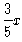. In particular, f(5) = 3 and f(1/5) = 3/25.

• The "My Dear Aunt Sally" interpretation (multiplication, division, addition, subtraction): Perform multiplication before division. For instance, the function f(x) = 3/5x gets interpreted as 3/(5x) =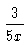. In particular, f(5) = 3/25 and f(1/5) = 3. Likewise, "ax/by" would be interpreted as "(ax)/(by)".

• The interpretation used by FORTRAN and some other computer languages (as well as some humans): Multiplication and division are given equal priority; a string of such operations is processed from left to right. For instance, "ax/by" would be interpreted as "((ax)/b))y", or more simply "(axy)/b".

Some students think that their electronic calculators can be relied upon for correct answers. But some calculators follow one convention, and other calculators follow another convention. In fact, some of the Texas Instruments calculators follow two conventions, according to whether multiplication is indicated by juxtaposition or a symbol:

• 3 / 5x is interpreted as 3/(5x), but
• 3 / 5 * x is interpreted as (3/5) x.
(Thanks to Chris Phillips and Thomas Cowdery for some of these examples and comments.)

Because there is no consensus of interpretation, I recommend that you do not write expressions like "3/5x" -- i.e., do not write a fraction involving a diagonal slash followed by a product, without any parentheses. Instead, use one of these four nonambiguous expressions: (3/5)x ,,   3/(5x),.

In some cases, additional information is evident from the context -- if one is familiar with the context. For instance, an experienced mathematician will recognize dy/dx as a derivative; it is the quotient of two differentials. The letter d represents the differential operator, not a variable. The expression dx represents the differential of x, not the product of two variables. Thus, parentheses are not needed, and would look rather strange if used. We do not write dy/(dx) or (dy)/(dx).

Here is another common error in the writing of fractions: If you write the horizontal fraction bar too high, it can be misread. For instance,orare acceptable expressions (with different meanings), but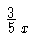is unacceptable -- it has no conventional meaning, and could be interpreted ambiguously as either of the previous fractions. I will not give full credit for ambiguous answers on any quiz or test. In this type of error, sloppy handwriting is the culprit. When you write an expression such as, be sure to write carefully, so that the horizontal bar is aimed at the middle of the x.

Here's one more example of interest. When entered as 2 ^ 3 ^ 4 without parentheses, the TI85 calculator shows 4096 and the TI89 shows 2.41785163923 E24. (Those are the answers to (2 ^ 3) ^ 4 and 2 ^ (3 ^ 4), respectively.) Thus, even the calculators made by one company don't all agree on their orders of operations. When in doubt, use parentheses! Thanks to Bill Dodge for this example.

Stream-of-consciousness equalities and implications. (My thanks to H. G. Mushenheim for identifying this type of error and suggesting a name for it.) This is an error in the intermediate steps in students' computations. It doesn't often lead to an erroneous final result at the end of that computation, but it is tremendously irritating to the mathematician who must grade the student's paper. It may also lead to a loss of partial credit, if the student makes some other error in his or her computation and the grader is then unable to decipher the student's work because of this stream-of-consciousness error.

To put it simply: Some students (especially college freshmen) use the equals sign (=) as a symbol for the word "then" or the phrase "the next step is." For instance, when asked to find the third derivative of x4+7x2–5, some students will write "x4+7x2–5 = 4x3+14x = 12x2+14 = 24x." Of course, those four expressions are not actually equal to one another.

A slight variant of this error consists of connecting several different equations with equal signs, where the intermediate equals signs are intended to convey "equivalent to" --- for example, x = y – 3 = x+3 = y. This is very confusing and altogether wrong, because equality is transitive --- i.e., if a=b and b=c then a=c, but x certainly is not equal to x+3. It would be better to replace that middle equals sign with some other symbol. The most obvious symbol for this purpose is ≡, which means "is equivalent to," but that symbol has the disadvantage of looking too much like an equals sign, and thus possibly leading to the same confusion. Thus, a better choice would be ↔ or ⇔, both of which mean "if and only if." Thus, I would rewrite the example above as x = y – 3 ⇔ x+3 = y.

There is also a more "advanced" form of this error. Some more advanced students (e.g., college seniors) use the implication symbol (⇒) as a symbol for the phrase "the next step is." A string of statements of the form

A ⇒ B ⇒ C ⇒ D
should mean that A by itself implies B, and B by itself implies C, and C by itself implies D; that is the coventional interpretation given by mathematicians. But some students use such a string to mean merely that if we start from A, then the next step in our reasoning is B (using not only A but other information as well) and then the next step is C (perhaps using both A and B), etc.

Actually, there is a symbol for "the next step is." It looks like this: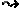It is also called "leads to," and in the LaTeX formatting language it is given by the code \leadsto. However, I haven't seen it used very often.

## Errors in Reasoning

Going over your work. Unfortunately, most textbooks do not devote a lot of attention to checking your work, and some teachers also skip this topic. Perhaps the reason is that there is no well-organized body of theory on how to check your work. Unfortunately, some students end up with the impression that it is not necessary to check your work -- just write it up once, and hope that it's correct. But that's nonsense. All of us make mistakes sometimes. In any subject, if you want to do good work, you have to work carefully, and then you have to check your work. In English, this is called "proofreading"; in computer science, this is called "debugging."

Moreover, in mathematics, checking your work is an important part of the learning process. Sure, you'll learn what you did wrong when you get your homework paper back from the grader; but you'll learn the subject much better if you try very hard to make sure that your answers are right before you turn in your homework.

It's important to check your work, but "going over your work" is the worst way to do it. I have twisted some words here, in order to make a point. By "going over your work" I mean reading through the steps that you've just done, to see if they look right. The drawback of that method is you're quite likely to make the same mistake again when you read through your steps! This is particularly true of conceptual errors -- e.g., forgetting to check for extraneous roots (discussed later on this web page).

You would be much more likely to catch your error if, instead, you checked your work by some method that is different from your original computation. Indeed, with that approach, the only way your error can go undetected is if you make two different errors that somehow, just by a remarkable coincidence, manage to cancel each other out -- e.g., if you arrive at the same wrong answer by two different incorrect methods. That happens occasionally, but very seldom.

In many cases, your second method can be easier, because it can make use of the fact that you already have an answer. This type of checking is not 100% reliable, but it is very highly reliable, and it may take very little time and effort.

Here is a simple example. Suppose that we want to solve 3(x–2)+7x = 2(x+1) for x. Here is a correct solution:

3(x–2)+7x = 2(x+1)
3x–6+7x = 2x+2
3x+7x–2x = 2+6
8x = 8
x = 1

Now, one easy way to check this work is to plug x = 1 into each side of the original equation, and see if the results come out the same. On the left side, we have 3(x–2)+7x = 3(1–2)+7(1) = 3(–1)+7(1) = (–3)+7 = 4. On the right side, we have 2(x+1) = 2(1+1) = 2(2) = 4. Those are the same, so the check works. It's easier than the original computation, because in the original computation we were looking for x; in the check, we already have a candidate for x. Nevertheless, this computation was by a different method than our original computation, so the answer is probably right.

Different kinds of problems require different kinds of checking. For a few kinds of problems, no other method of checking besides "going over your work" will suggest itself to you. But for most problems, some second method of checking will be evident if you think about it for a moment.

If you absolutely can't think of any other method, here is a last-resort technique: Put the paper away somewhere. Several hours later (if you can afford to wait that long), do the same problems over -- by the same method, if need be -- but on a new sheet of paper, without looking at the first sheet. Then compare the answers. There is still some chance of making the same error twice, but this method reduces that chance at least a little. Unfortunately, this technique doubles the amount of work you have to do, and so you may be reluctant to employ this technique. Well, that's up to you; it's your decision. But how badly do you want to master the material and get the higher grade? How much importance do you attach to the integrity of your work?

When you do find that your two answers differ, work very carefully to determine which one (if either) is correct. Don't hurry through this crucial last part of the process. You've already demonstrated your fallibility on this type of problem, so there is extra reason to doubt the accuracy of any further work on this problem; check your results several times.

Perhaps the error occurred through mere carelessness, because you weren't really interested in the work and you were in a hurry to finish it and put it aside. If so, don't compound that error. You now must pay for your neglect -- you now must put in even more time to master the material properly! The problem won't just go away or lose importance if you ignore it. Mathematics, more than any other subject, is vertically structured: each concept builds on many concepts that preceded it. Once you leave a topic unmastered, it will haunt you repeatedly throughout many of the topics that follow it, in all of the math courses that follow it.

Also, if discover that you've made an error, try to discover what the error was. It may be a type of error that you are making with some frequency. Once you identify it, you may be better able to watch out for it in the future.

Not noticing that some steps are irreversible. If you do the same thing to both sides of a true equation, you'll get another true equation. So if you have an equation that is satisfied by some unknown number x, and you do the same thing to both sides of the equation, then the new equation will still be satisfied by the same number x. Thus, the new equation will have all the solutions x that the old equation had -- but it might also have some new solutions.

Some operations are reversible -- i.e., we have the same set of solutions before and after the operation. For instance,

• The operation "multiply both sides of the equation by 2" is reversible. For example, the set of all numbers x that satisfy x2–3x–2 = –4 is the same as the set of all numbers x that satisfy 2x2–6x–4 = –8. In fact, to reverse the operation, we just have to multiply both sides of an equation by 1/2.
• The operation "subtract 7 from both sides" is reversible. To reverse it, just add 7 to both sides.
Some operations are not reversible, and so we may get new solutions when we perform such an operation. For instance,
• The operation "square both sides" is not reversible. For instance, the equation x = –3 has only one solution, but when we square both sides, we get x2 = 9, which has two solutions.
• The operation "multiply both sides by x–4" is not reversible. The resulting equation will have for its solutions all of the solutions of the original equation plus the additional new solution x=4.

A commonly used method for solving equations is this: Construct a sequence of equations, going from one equation to the next by doing the same thing to both sides of an equation, choosing the operations to gradually simplify the equation, until you get the equation down to something obvious like "x=5". This method is not bad for discovery, but as a method of certification it is unreliable. To make it reliable, you need to add one more rule:

 if any of your steps are irreversible, then you must check for extraneous roots when you get to the end of the computation.

That's because, at the end of your computational procedure, you'll have not only the solution(s) to the original problem, but possibly also some additional numbers that do not solve the original problem. How do you check for them? Just plug each of your answers into the original problem, to see whether it works. Many students, unfortuntely, omit that last step.

First example:

The given problem is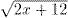– 2 = x. To begin solving this problem, add 2 to both sides (a reversible step); we obtain= x + 2. Square both sides -- an irreversible step; we obtain 2x+12 = x2+4x+4. By adding and subtracting appropriate amounts to both sides of the equation (a reversible step), we obtain x2+2x–8 = 0. Now solve that quadratic equation by your favorite method -- by the quadratic formula, by completing the square, or by factoring by inspection. We obtain

x = 2 or x = – 4.

Unfortunately, many students stop at this step; they believe they're done; they write {2, –4} for their answer. A correct solution continues as follows: Since at least one of the steps in our procedure was irreversible, we must check for extraneous roots. Check each of the numbers 2 and –4, to see if it satisfies the equation originally given in the problem.

• When x=2, then– 2 = (4+12)1/2 – 2 = 4 – 2 = x, so we have a correct solution. However,
• When x= – 4, then– 2 = (–8+12)1/2 – 2 = 2 – 2 ≠ x, so we have an incorrect solution.

Thus the correct answer is {2}.

Second example.
The given problem is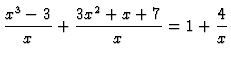. By adding and subtracting (reversible), we obtain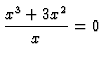. By factoring (reversible), we obtain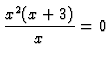. Cancel out an x (irreversible); we obtain x(x+3)=0. The solutions appear to be x=0 and x = –3. Some students unfortunately stop here, but we shouldn't -- one of our steps was irreversible. Checking reveals that x=0 doesn't work in the given problem, so it's extraneous. The correct answer is just x = –3.

Of course, even aside from the issue of extraneous roots, another reason to check your answers is to avoid arithmetic errors. This is a special case of "checking your work," mentioned elsewhere on this web page. We all make computational mistakes; we can catch most of our computational mistakes with a little extra effort.

The extraneous roots error was brought to my attention by Dr. Richard Beldin. Professor Beldin tells me that he gave a test heavily laced with extraneous roots problems, and warned the students that

• such problems would appear on the test, and
• the appearance of an extraneous root in an answer would cost half the credit for a problem, so
• the students should check for extraneous roots.
Professor Beldin reports that, nevertheless, about a third of the students neglected to check, on so many problems that they lost two letter grades on the overall the test score.

Professor Stephen Glasby reports this interesting example of ignoring irreversibility. We wish to prove sin x = √(1 - cos2 x). We begin by squaring both sides of that equation; we obtain sin2 x = 1 - cos2 x. Rearrange terms to obtain sin2 x + cos2 x = 1. That's true, so apparently the proof is done. But it's not, because squaring both sides was irreversible. In fact, the equation sin x = √(1 - cos2 x) that we've just "proved" isn't true -- for instance, try x = - π/2.

Confusing a statement with its converse. The implication "A implies B" is not the same as the implication "B implies A." For instance,

if I went swimming at the beach today, then I got wet today
is a true statement. But
if I got wet today, then I went swimming at the beach today
doesn't have to be true -- maybe I got wet by taking a shower or bath at home. The difference is easy to see in concrete examples like these, but it may be harder to see in the abstract setting of mathematics.

Some technical terminology might be helpful here. The symbol ⇒ means "implies." The two statements "A ⇒ B" and "B ⇒ A" are said to be converses of each other. What we've just explained is that an implication and its converse generally are not equivalent.

I should emphasize the word "generally" in the last paragraph. In a few cases the implications "A ⇒ B" and "B ⇒ A" do turn out to be equivalent. For instance, let p,q,r be the lengths of the sides of a triangle, with r being the longest side; then

p2+q2=r2   if and only if   the triangle is a right triangle.
The "if" part of that statement is the well-known Pythagorean Theorem; the "only if" part is its converse, which also happens to be true but is less well known.

Some students confuse a statement with its converse. This may stem partly from the fact that, in many nonmathematical situations, a statement is equivalent to its converse, and so in everyday "human" English we often use the word "if" interchangeably with the phrase "if and only if". For instance,

I'll go to the vending machine and buy a snack if I get hungry
sounds reasonable. But most people would figure that if I do not get hungry, then I won't go buy a snack. So, evidently, what I really meant was
I'll go to the vending machine and buy a snack if and only if I get hungry.
Most people would just say the shorter sentence, and mean the longer one; it's a sort of verbal shortcut. Generally you can figure out from the context just what the real meaning is, and usually you don't even think about it on a conscious level.

To make matters more confusing, mathematicians are humans too. In certain contexts, even mathematicians use "if" when they really mean "if and only if." You have to figure this out from the context, and that may be hard to do if you're new to the language of mathematics, and not a fluent speaker. Chiefly, mathematicians use the verbal shortcut when they're giving definitions, and then you have a hint: the word being defined usually is in italics or boldface. For instance, here is the definition of continuity of a real-valued function f:

f is continuous if for each real number p and each positive number ε there exists a positive number δ (which may depend on p and ε) such that, for each real number q, if | p - q | < δ, then | f(p) - f(q) | < ε.
The fourth word in this very long sentence is an "if" that really means "if and only if", but we know that because "continuous" is in boldface; this is the definition of the word "continuous".

Converses also should not be confused with contrapositives. Those two words sound similar but they mean very different things. The contrapositive of the implication "A ⇒ B" is the implication "(~B) ⇒ (~A)", where ~ means "not." Those two statements are equivalent. For instance,

if I went swimming at the beach today, then I got wet today
has exactly the same meaning as the more complicated sounding statement
if I didn't get wet today, then I didn't go swimming at the beach today.
Sometimes we replace a statement with its contrapositive, because it may be easier to prove, even if it is more complicated to state. (Thanks to Valery Mishkin for bringing this class of errors to my attention.)

Working backward. This is an unreliable method of proof used, unfortunately, by many students. We start with the statement that we want to prove, and gradually replace it with consequences, until we arrive at a statement that is obviously true (such as 1 = 1). From that some students conclude that the original statement is true. They overlook the fact that some of their steps might be irreversible.

Here is an example of a successful and correct use of "working backward": we are asked to prove that the cube root of 3 is greater than the square root of 2. We write these steps:

• Start by assuming the thing that we're trying to prove: 31/3 > 21/2.
• Raise both sides to the power 6; that yields (31/3)6 > (21/2)6.
• Simplify both sides, using the rule of exponents that says (ab)c = a(bc). Thus we obtain 32 > 23.
• Evaluate. That yields 9 > 8, which is clearly true.
Some students would believe that we have now proved 31/3 > 21/2. But that's not a proof -- you should never begin a proof by assuming the very thing that you're trying to prove. In this example, however, all the steps happen to be reversible, so those steps can be made into a proof. We just have to rewrite the steps in their proper order:
• 9 > 8 is obviously true.
• Rewrite that as 32 > 23.
• Rewrite that as (31/3)6 > (21/2)6, by using the rule of exponents that says (ab)c = a(bc).
• Now take the sixth root on both sides. That leaves 31/3 > 21/2.

Working backward can be a good method for discovering proofs, though it has to be used with caution, as discussed below. But it is an unacceptable method for presenting proofs after you have discovered them. Students must distinguish between discovery (which can be haphazard, informal, illogical) and presentation (which must be rigorous). The reasoning used in working backward is a reversal of the reasoning needed for presentation of the proof -- but that means replacing each implication "A ⇒ B" with its converse, "B ⇒ A". As we pointed out a few paragraphs ago, those two implications are sometimes not equivalent.

In some cases, the implication is reversible -- i.e., some reversible operation (like multiplying both sides of an equation by 2, or raising both sides of an inequality to the sixth power when both sides were already positive) transforms statement A into statement B. Perhaps the students have gotten into the habit of expecting all implications to be reversible, because early in their education they were exposed to many reversible transformations -- adding three, multiplying by a half, etc. But in fact, most implications of mathematical statements are not reversible, and so "working backward" is almost never acceptable as a method of presenting a proof.

Working backward can be used for discovering a proof (and, in fact, sometimes it is the only discovery method available), but it must be used with appropriate caution. At each step in the discovery process, you start from some statement A, and you create a related statement B; it may be the case that the implication A implies B is obvious. But you have to think about whether B implies A. If you can find a convincing demonstration that B implies A, then you can proceed. If you can't find a demonstration of B implies A, then you might as well discard statement B, because it is of no use at all to you; look for some other statement to use instead.

Beginners often make mistakes when they use "working backward," because they don't notice that some step is irreversible. For instance, the statement x >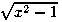is not true for all real numbers x. But if we didn't know that, we might come up with this proof:

• Start with what we want to prove: x >.
• Sincemeans the nonnegative square root of x2–1, we know that it is nonnegative. Since x is even larger, it is also nonnegative.
• Squaring both sides of an inequality is a reversible step if both sides are nonnegative. Thus we obtain x2 > x2–1.
• Subtract x2 from both sides; 0 > –1. And that's clearly true, regardless of what x is.
• "Therefore x >for all real numbers x."
But that conclusion is wrong. The right side of the inequality is undefined when x = 0.5. And when x = –2, then both sides of the inequality are defined, but the inequality is false. See if you can find where the reasoning went awry.

Well then, if reasoning backward is not acceptable as a presentation of a proof, what is acceptable? A direct proof is acceptable. A theorem has certain hypotheses (assumptions) and certain conclusions. In a direct proof, you start with the hypotheses, and you generate consequences -- i.e., you start making sentences, where each sentence is either a hypothesis of the theorem, an axiom (if you're using an abstract theory), or a result deduced from some earlier theorem using sentences you've already generated in the proof. They must be in order -- i.e., if one sentence A is used to deduce another sentence B, then sentence A should appear before sentence B. The goal is to eventually generate, among the consequences, the conclusion of the desired theoreom.

Some variants on this are possible, but only if the explanatory language is used very carefully; such variants are not recommended for beginners. The variants involve phrases like "it suffices to show that...". These phrases are like foreshadowing in a story, or like direction signs on a highway. They intentionally appear out of chronological order, to make the intended route more understandable. But in some sense they are not really part of the official proof; they are just commentaries on the side, to make the official proof easier to understand. When you pass a sign that says "100 miles to Nashville," you're not actually in Nashville yet.

Perhaps the biggest failure in the proofs of beginners is a severe lack of words. A beginner will write down an equation that should be accompanied by either the phrase "we have now shown" or the phrase "we intend to show", to clarify just where we are in the proof. But the beginner writes neither phrase, and the reader is expected to guess which it is. This is like a novel in which there are many flashbacks and also much foreshadowing, but all the verbs are in present tense; the reader must try to figure out a logical order in which the events actually occur.

One easy method that I have begun recommending to students is this: Put a questionmark over any relationship (equals sign, greater than sign, etc.) that represents an assertion that you want to prove, but have not yet proved. An equals-sign without a questionmark will then be understood to represent an equation that you have already proved. Later you can put a checkmark next to the equations whose doubt has been removed. This method may help the student writing the work, but unfortunately it does not greatly help the teacher or grader who is reading the work -- the order of steps is still obscured.

Another common style of proof is the indirect proof, also known as proof by contradiction. In this proof, we start with the hypotheses of the desired theorem; but we may also add, as additional hypothesis, the statement that "the desired theorem's conclusion is false." In other words, we really want to prove A ⇒ B, so we start by assuming both A and ~B (where ~ means "not"). We then start generating consequences, and we try to generate a contradiction among our consequences. When we do so, this establishes that A ⇒ B must have been true after all. This kind of proof is harder to read, but it is actually easier to discover and to write: we have more hypotheses (not only A, but also ~B), so it is easier to generate consequences. I recommend that beginners avoid indirect proofs as long as possible; but if you continue with your math education, you will eventually run into some abstract theorems in higher math that can only be proved by indirect proof.

Difficulties with quantifiers. Quantifiers are the phrases "there exists" and "for every." Many students -- even beginning graduate students in mathematics! -- have little or no understanding of the use of quantifiers. For instance, which of these statements is true and which is false, using the standard real number system?

For each positive number a there exists a positive number b such that b is less than a.

There exists a positive number b such that for each positive number a we have b less than a.

Difficulty with quantifiers may be common, but I'm not sure what causes the difficulty. Perhaps it is just that mathematical sentences are grammatically more complicated than nonmathematical ones. For instance, a real-valued function f defined on the real line is continuous if
for each point p and for each number epsilon greater than zero, there exists a number delta greater than zero such that, for each point q, if the distance from p to q is less than delta, then the distance from f(p) to f(q) is less than epsilon.
This sentence involves several nested clauses, based on several quantifiers:
• for each point p ...
• for each number epsilon ...
• there exists delta such that ...
• for each point q ...
Nonmathematical grammar generally doesn't involve so many nested clauses and such crucial attention to the order of the words.

I think that many students would benefit from thinking of quantifiers as indicators of a competition between two adversaries, as in a court of law. For instance, when I assert that the function f is continuous, I am asserting that

no matter what point p and what positive number epsilon you specify, I can then specify a corresponding positive number delta, such that, no matter what point q you then specify, if you demonstrate that your q has distance from your p less than my delta, then I can demonstrate that the resulting f(p) and f(q) are separated by a distance less than your epsilon.
Of course, it must be understood that the two adversaries in mathematics are emotionally and morally neutral. In a court of law (at least, as depicted on television), it is often the case that one side is the "good guys" and the other side is the "bad guys," but in principle the law is supposed to be a neutral way of seeking the truth; mathematical reasoning is too.

Some students may have an easier time avoiding errors with quantifiers if they actually use symbols instead of words. This may make the differences in the quantifiers more visually prominent. The symbols to use are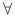universal quantifier "for all" (or "for each")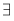existential quantifier "there exists" (or "there exists at least one")
With those symbols, my earlier two statements about real numbers can be written, respectively, as
• (a > 0) (b > 0) (b < a).
• (b > 0) (a > 0) (b < a).
And the definition of continuity of a real-valued function f defined on the real line can be restated as
(p) (ε>0) (δ>0) (q) (if |p–q|<δ, then |f(p)–f(q)|<ε).
Now you can see the four nested quantifiers very clearly; this may explain why the definition is so complicated -- and perhaps it will help to clarify what the definition means.

Some readers have requested that I add a few words about negations of quantifiers. The basic rules are these: ~=~ and ~=~, where ~ means "not". That is, you can move a negation past a quantifier, if you just switch which type of quantifier you're using. An example of ~=~:

Saying "not every peanut in this jar is stale" is the same thing as saying "at least one peanut in this jar is not stale."
An example of ~=~:
Saying "there does not exist a stale peanut in this jar" is the same thing as saying "every peanut in this jar is non-stale."
Here is a more complicated example: Following are a few different ways to say that "f is not continuous". Start with the formula that I gave above, but with a "not" in front of it. Gradually move the "not" to the right, switching each quantifier that it passes. So all these statements are equivalent:
• ~ (p) (ε>0) (δ>0) (q) (if |p–q|<δ, then |f(p)–f(q)|<ε).
• (p) ~ (ε>0) (δ>0) (q) (if |p–q|<δ, then |f(p)–f(q)|<ε).
• (p) (ε>0) ~ (δ>0) (q) (if |p–q|<δ, then |f(p)–f(q)|<ε).
• (p) (ε>0) (δ>0) ~ (q) (if |p–q|<δ, then |f(p)–f(q)|<ε).
• (p) (ε>0) (δ>0) (q) ~ (if |p–q|<δ, then |f(p)–f(q)|<ε).
• (p) (ε>0) (δ>0) (q) (|p–q|<δ but |f(p)–f(q)|≥ε).

Erroneous method justified by one or two instances of correct results. Sometimes an erroneous method can lead (just by coincidence) to a correct result. But that does not justify the method.

Sample problem: Simplify 16/64.
Erroneous method: Cancel the 6's.
Below is another computation like that. Can you find all of its errors? Some student actually turned this in on an exam, and expected partial credit because he had the right answer. (Thanks to Sean Raleigh for bringing this one to my attention; the solution was graded by Boern Lamel.)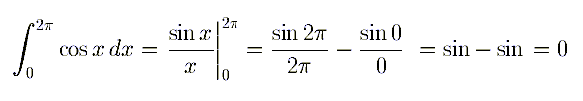Unquestioning faith in calculators. Many students believe that their calculators are always right. But that is not true. All calculators have limitations, and will give incorrect answers under some circumstances (as will math teachers and math books).

Probably the most common error with calculators is simply forgetting to switch between degrees and radians (or not understanding the need to switch). Degrees are often used in engineering and science classes, but radians are almost always used in calculus and higher math classes. That's because most of the formulas involving trigonometric functions come out much simpler with radians than with degrees -- the formulas for the derivatives, for the power series expansions, etc.

Here is another widely occurring calculator error. Some graphing calculators, if asked to display a graph of x^(1/3) or x^(2/3), will only display the right half of the graph -- i.e., there will be no points plotted in the left half-plane. But the function x^(1/3) is odd, and the function x^(2/3) is even; both functions (if graphed correctly) have points in both the right and left half-planes. To get a correct graph, you need to look in the calculator's function menus until you find a special "button" for cube roots. Use that to get x^(1/3); use the square of the cube root to get x^(2/3).

Why is that? Well, first you need to understand that for some constants k, the correct graph of x^k is blank in the left half-plane, because the function x^k is actually undefined for x < 0. For instance, k = 1/2 is one such constant. The numbers 1/3 and 2/3 are not such constants, but if you simply punch in the formula x^(1/3) or x^(2/3) using the caret symbol (^) for exponentiation, the calculator must replace the fractions 1/3 or 2/3 with some sort of approximations such as k=0.3333 or k=0.6667. Those approximations turn out to have the same property I just mentioned for k=0.5 -- the resulting function is undefined for x less than 0. You avoid this approximation error by using the cube root button.

Dave Rusin has put together some notes on the wide variety of errors one can make by not understanding one's calculator. By the way, I'll take this opportunity to mention that Dave Rusin has put together a super website, Mathematical Atlas: A gateway to Mathematics, which offers definitions, introductions, and links to all sorts of topics in math.

## Unwarranted Generalizations

A formula or notation may work properly in one context, but some students try to apply it in a wider context, where it may not work properly at all. Robin Chapman also calls this type of error "crass formalism." Here is one example that he has mentioned:

Every positive number has two square roots: one positive, the other negative. The notation √b generally is only used when b is a nonnegative real number; it means "the nonnegative square root of b," and not just "the square root of b." The notation √b probably should not be used at all in the context of complex numbers. Every nonzero complex number b has two square roots, but in general there is no natural way to say which one should be associated with the expression √b. The formula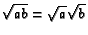is correct when a and b are positive real numbers, but it leads to errors when generalized indiscriminately to other kinds of numbers. Beginners in the use of complex numbers are prone to errors such as. In fact, the great mathematician Leonhard Euler published a computation similar to this in a book in 1770, when the theory of complex numbers was still young.

Here is another example, from my own teaching experience: What is the derivative of xx? If you ask this during the first week of calculus, a correct answer is "we haven't covered that yet." But many students will very confidently tell you that the answer is x • xx–1. Some of them may even simplify that expression -- it reduces to xx -- and a few students will even remark: "Say, that's interesting -- xx is its own derivative!" Of course, all these students are wrong. The correct answer, covered after about a semester of calculus, is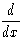(xx) = xx (1 + ln x).

The difficulty is that, in high school or shortly after they arrive at college, the students have learned that(xk) = kxk–1

That formula is actually WRONG, but in a very subtle way. The correct formula is(xk) = kxk–1 (for all x where the right side is defined), if k is any constant.

The equation is unchanged, but it's now accompanied by some words telling us when the equation is applicable. I've thrown in the parenthetical "for all x where the right side is defined," in order to avoid discussing the complications that arise when x ≤ 0. But the part that I really want to discuss here is the other part -- i.e., the phrase "if k is any constant."

To most teachers, that additional phrase doesn't seem important, because in the teacher's mind "x" usually means a variable and "k" usually means a constant. The letters x and k are used in different ways here, a little like the difference between bound and free variables in logic: Fix any constant k; then the equation states a relationship between two functions of the variable x. So the language suggests to us that x is probably not supposed to equal k.

But the math teacher is already fluent in this language, whereas mathematics is a foreign language to most students. To most students, the distinction between the two boxed formulas is one which doesn't seem important at first, because the only examples shown to the student at first are those in which k actually is a constant. Why bother to mention that k must be a constant, when there are no other conceivable meanings for k? So the student memorizes the first (incorrect) formula, rather than the second (correct) formula.

Every mathematical formula should be accompanied by a few words of English (or your natural language, whatever it is). The words in English tell when the formula can or can't be applied. But frequently we neglect the words, because they seem to be clear from the context. When the context changes, the words that we've omitted may become crucial.

Students have difficulty with this. Here is an experiment that I have tried a few times: At the beginning of the semester, I tell the students that the correct answer to(xx) is not xx, but rather xx (1 + ln x), and I tell them that this problem will be on their final exam at the end of the semester. I repeat these statements once or twice during the semester, and I repeat them again at the very end of the semester, just before classes end. Nevertheless, a large percentage (sometimes a third) of my students still get the problem wrong on the final exam! Their original, incorrect learning persists despite my efforts.

I have a couple of theories about why this happens: (i) For most students, mathematics is a foreign language, and the student focuses his or her attention on the part which seems most foreign -- i.e., the formulas. The words have the appearance of something familiar ("oh, that's just English, and I already know English"), and so the student doesn't pay a lot of attention to the words. (ii) Undergraduate students tend to focus on mechanical computations; they are not yet mathematically mature enough to be able to think easily about theoretical and abstract ideas.

A sort of footnote: Here is a common error among readers of this web page. Several people have written to me to ask, shouldn't that formula say "if k is any constant except 0", or "if k is any constant except –1", or something like that? They think some special note needs to be made about the logarithm case. Actually, my formula is correct as it stands -- i.e., for every constant real number k -- but if you want to tell the whole story, you'd have to append some additional formula(s). When k=0, my formula just says the derivative of 1 is 0x–1; that's true but not very enlightening. My formula doesn't mention, but also doesn't contradict, the fact that the derivative of ln(x) is 1x–1. You can always say more about any subject, but I just wanted to contrast the formulas xk and xx as simply as possible. ... And of course, for simplicity's sake, I haven't mentioned the complications you run into when x is zero or a negative number; I'm only considering those values of x for which xk and xx are easy to define.

## Other Common Calculus Errors

Jumping to conclusions about infinity. Some problems involving infinity can be solved using "the elementary arithmetic of infinity". Some students jump to the conclusion that all problems involving infinity can be solved by this sort of "elementary arithmetic," and so they guess all sorts of incorrect answers (mainly 0 or infinity) to such problems.

Here is an example of the "elementary arithmetic": If we use the equation cautiously, we can say (informally) that 1/∞ = 0 -- though perhaps it would be less misleading to write instead 1/∞ → 0. (My thanks to Hans Aberg for this suggestion and for several other suggestions on this web page.) What this rule really means is that if you take a medium-sized number and divide it by an enormous number, you get a number very close to 0. For instance, without doing any real work, we can use this rule to conclude at a glance that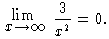Thus, the problem 1/∞ has the answer 0. The problem ∞ – ∞ does not have an answer in any analogous fashion; we might say that ∞ – ∞ is undefined. This does not mean that "Undefined" is the answer to any problem of the form ∞ – ∞. What it means, rather, is that each problem involving ∞ – ∞ requires a separate analysis; different problems of this type have different answers. For instance,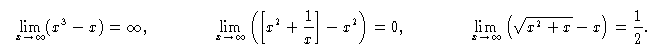Those first two problems are fairly obvious; the last problem takes more sophisticated analysis. Just guessing would not get you an answer of 1/2. (If you don't understand what is going on in the last problem, try graphing the functions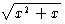and x on one display screen on your graphing calculator. That may provide a lot of insight, though it's not a proof.)

In a similar fashion,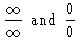do not have quick and easy answers; they too require more specialized and sophisticated analyses.

Here is a common error mentioned by Stuart Price: Some students seem to think that limn→∞ (1+(1/n))n = 1. Their reasoning is this: "When n→∞, then 1+(1/n) → 1. Now compute limn→∞ 1n = 1." Of course, this reasoning is just a bit too simplistic. You have to deal with both of the n's in the expression (1+(1/n))n at the same time -- i.e., they both go to infinity simultaneously; you can't figure that one goes to infinity and then the other goes to infinity. And in fact, if you let the other one go to infinity first, you'd get a different answer: limn→∞ (1+0.0000001)n = ∞. So evidently the answer lies somewhere between 1 and ∞. That doesn't tell us much; my point here is that easy methods do not work on this problem. The correct answer is a number that is near 2.718. (It's an important constant, known to mathematicians as "e".) There's no way you could get that by an easy method.

That reminds me of a related question that seems to bother many students: What is 00 ?

The reason that a question arises at all is because xy is discontinuous at (0,0). Indeed, we have x0=1 for all x>0, and we have 0y=0 for all y>0. And limx,y → 0+ xy doesn't exist, because that expression means the limit of xy as the point (x,y) approaches (0,0) along all paths where xy is defined.

Nevertheless, many (most?) mathematicians will define 00 = 1, just for convenience, because that makes the most formulas work (and then they will note exceptions for formulas that require a different definition).

For instance, if we're working with polynomials or power series,

p(x) = a0x0 + a1x1 + a2x2 + a3x3 + ... + anxn + ...

-- perhaps the most common place for 00 to arise -- then it's convenient to have 00 = 1, since a0x0 needs to be equal to a0. The Binomial Theorem would be more complicated to write if we defined 00 any other way.

Problems with series. Sean Raleigh reports that the most common series error he has seen is this: If a1, a2, a3, ... is a sequence converging to 0, then many students conclude (erroneously) that the series a1 + a2 + a3 + ... must be convergent (i.e., must add up to a finite number). Perhaps they hold that belief because it is true for most of the examples that they have seen. Most counterexamples are too advanced to be included in an elementary textbook. Of course, every calculus book gives the simple example of the harmonic series:

1 + (1/2) + (1/3) + (1/4) + ...   =   ∞
but one single example of divergence does not seem to outweigh in the students' minds the many examples of convergence that they have seen.

Loss or misuse of constants of integration. The indefinite integral of a function involves an "arbitrary constant", and this causes confusion for many students, because the notation doesn't convey the concept very well. An expression such as "3x2+5x+C" really is supposed to represent an infinite collection of functions -- it represents all of the functions

3x2+5x+7, 3x2+5x+19, 3x2+5x–3.19, etc.
plus more functions of the same sort. One of the difficulties, also, is that the same letter "C" is customarily used for all such arbitrary constants; but one computation may involve several different arbitrary constants. It would be more accurate to put subscripts on the C's, to differentiate one of them from another -- i.e., write C1, C2, C3, etc. -- and I often do that in my lectures.

Here is an example. The formula for Integration By Parts, in its briefest form, is ∫udv = uv - ∫vdu; that can be understood more easily as

 ∫u(x)v'(x)dx = u(x)v(x) - ∫u'(x)v(x)dx.

Now, that formula is correct, but it can easily be mishandled and can lead to errors. Here is one particularly amusing error: Plug u(x)=1/x and v(x)=x into the formula above. We get

 ∫(1/x)(1)dx = (1/x)(x) - ∫(-1/x2)(x)dx

which simplifies to

(1/x)dx = 1 + (1/x)dx.

Now, regardless of what you think is the value of (1/x)dx, you just have to subtract that amount from both sides of the preceding equation, to obtain 0=1. Wait, how can that be???? Well, if we're very careful, we realize that the two (1/x)dx's on the two sides of the last equation are not actually the same. What that last equation really says is

[ln|x| + C1] = 1 + [ln|x| + C2].

That is a true equation, if we choose the constants C1 and C2 appropriately -- i.e., if we choose them so that C1–C2=1. Thus, the two constants are not independent of each other -- they are not completely "arbitrary". Perhaps a more accurate explanation is this: The two expressions [ln|x| + C1] and 1+[ln|x| + C2] do not actually represent individual functions; rather, each of those expressions represents a set of functions.

• The expression [ln|x| + C1] represents the set of all the functions of x that can be obtained by starting with the function ln|x| and then adding a constant.

• The expression 1 + [ln|x| + C2] represents the set of all the functions of x that can be obtained by starting with the function ln|x|, then adding a constant, and then adding 1.
Those two descriptions may sound different, but if you think about it, you'll see that those descriptions are nevertheless specifying the same set. My thanks to Antonio Ferraioli ("Ferra") for this 0=1 paradox and its explanation.

Some students manage to make this kind of error even with definite integrals. They start from the formula (1/x)dx = 1 + (1/x)dx, which is correct; but then when they "switch to definite integrals", they get the formula ab (1/x)dx = 1 + ab (1/x)dx, which is not correct. If you really want to "switch to definite integrals", you need to think of that constant 1 as a special sort of function. When you switch to definite integrals, any function p(x) gets replaced by p(b)–p(a). In particular, the constant function 1 is the function given by p(x)=1 for all x. So p(b)–p(a) becomes 1–1, or 0.

Some students may understand this better if we do the whole thing with definite integrals, right from the start. Let's use the formula

 ∫ab u(x)v′(x)dx = u(b)v(b) – u(a)v(a) – ∫ab u′(x)v(x)dx.

Note that this formula has one more term than my previous boxed formula -- when we convert u(x)v(x) to the definite integral version, we replace it with u(b)v(b)–u(a)v(a). Now plug in u(x)=1/x and v(x)=x. We get

ab (1/x)(1)dx = (1/b)(b) – (1/a)(a) – ab (–1/x2)(x)dx

which (assuming 0 is not in the interval [a,b]) simplifies to

[ln|b|–ln|a|] = 1 – 1 – [ln|b|+ln|a|]

which is true -- i.e., there is no contradiction here.

Some students may be puzzled by the differences between the two versions of the Integration by Parts formula (in boxes, in the last few paragraphs). I will describe in a little more detail how you get from the definite integral formula (in the last box) to the indefinite integral formula (in the first box in this section). Think of a as a constant and b as a variable, and you'll get something like this:

[ u(x)v′(x)dx + C1] = [u(x)v(x) – C2][ u′(x)v(x)dx + C3].

Note that the u(b)v(b) term gets replaced by u(x)v(x), and the u(a)v(a) term "disappears" because it is constant. Finally, we can "absorb" the arbitrary constants into the indefinite integrals -- i.e., we don't need to write C1, C2, C3, because any indefinite integral is only determined up to adding or subtracting a constant anyway. Thus, we arrive at the briefer formula u(x)v′(x)dx = u(x)v(x) – u′(x)v(x)dx.

Handling constants of integration gets even more complicated in the first course on differential equations, and there are even more kinds of errors possible. I won't try to list all of them here, but here is the simplest and most common error that I've seen: In calculus, some students get the idea that you can just omit the "+C" in your intermediate computations, and then tack it on at the end of your answer, if you know which kinds of problems require an arbitrary constant. That will usually work in calculus, but it doesn't work in differential equations, because in differential equations the "C" can show up anywhere -- not necessarily as a "+C" at the end of the answer.

Here's a simple example: Let's solve the differential equation xy′+7=y (where y′ means dy/dx). One way to solve it is by the following steps:

• Rewrite the problem as y′ – (1/x)y = – 7/x, to show that it is linear.

• The integrating factor is then exp[ (–1/x)dx] = 1/x.

• Multiply both sides of the differential equation by the integrating factor, to obtain an exact differential equation: (y/x)′ = (1/x)y′ – (1/x2)y = –7/x2.

• Integrate both sides. Thus y/x = (7/x) + C.

• Solve for y. Thus y = 7 + Cx.
That's the correct answer. But if we had taken the attitude "don't bother with C, just tack it on when you're done," instead of the last two steps we'd have written:
• "Integrate both sides. Thus y/x = 7/x."

• "Solve for y. Thus y = 7. "

• "Tack on the "+C". Thus y = 7 + C. "
That's wrong, whether you simplify it or not.

Loss of differentials. This shows up both in differentiation and in integration. The "loss of differentials" is much like the "loss of invisible parentheses" discussed earlier in this document; it is a type of sloppy writing in intermediate steps which leads to actual errors in the final answer.

When students first begin to learn to differentiate, they are always differentiating with respect to the same variable, and so they see no reason to mention that variable. Thus, in differentiating the function y = f(x) = 7x3+5x, they may correctly write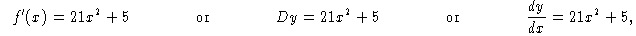or they may incorrectly write "dy = 21x2+5." The omission of the "dx" from this last equation makes no real difference in the student's mind, and this slovenly omission may become a habit. But it will cause difficulties later in the course. In fact, I am starting to think that we could avoid a lot of difficulty if we discourage beginning calculus students from using the notations f ′(x) or Dy. If we require them to use the notation dy/dx , and penalize them for writing it as dy, we might save them a lot of headaches later.

The difficulty, of course, shows up when we arrive at the Chain Rule. Suddenly, the question is no longer "What is the derivative of y", but rather, "What is the derivative of y with respect to x? with respect to u? How are those two derivatives related?" The student who does not make a habit of distinguishing between dy/dx and dy/du in writing may also have difficulty distinguishing between them conceptually, and thus will have difficulty understanding the Chain Rule.

This also leads to difficulties with the "u-substitutions" rule, which is just the Chain Rule turned into a rule about integrals. For instance: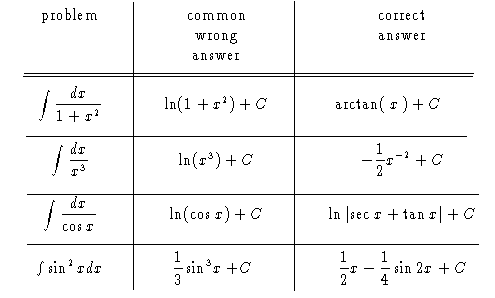What causes these errors?

For the first three problems, the student is attempting to use the formula  (1/u)du = ln |u|+C (which is a correct formula, but not directly applicable). However, the student has learned it incorrectly as  "  (1/u) = ln |u|+C." Substitute u = 1+x2 or u = x3 or u = cos x into that formula to get the first three erroneous answers in the table above. The expressions  (1/u)du and  (1/u)dx have very different meanings, but you're likely to confuse them if you write them both as  (1/u).

For the last problem in the table above, the student is attempting to use the formula ∫ u2du = (1/3)u3+C, which is a correct formula, but not relevant to the present problem. The student has probaby memorized that formula in the incorrect form ∫ u2 = (1/3)u3+C. The expressions ∫ u2du and ∫ u2dx have very different meanings, but you're likely to confuse them if you write them both as ∫ u2.

Another correct way to write the rule about logarithms is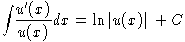. Since this expresses everything in terms of the variable x, it may make errors less likely. Admittedly, it is a complicated looking formula, but it is preferable to a wrong formula. The first, third, and fourth problems in the preceding table all require more complicated methods; just using logarithms won't solve the problems for you. The problem of integrating x –3 actually requires a less complicated method -- i.e., without logarithms.

We should prohibit students from writing an integral sign without a matching differential. Just as any "(" must be matched with a ")", so too any integral sign must be matched with a "dx" or "du" or "dt" or whatever. The expression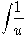is unbalanced, and should be prohibited. If we're considering a substitution of u = 1+x2, then  (1/u)du is very different from  (1/u)dx, and so the expression  (1/u) is ambiguous and meaningless. If you write  (1/u) in one of your intermediate steps, you may forget whether it represents  (1/u)du or  (1/u)dx, and you may inadvertently switch from one to the other -- thus replacing one mathematical quantity with another to which it is not equal.

By the way, some students get confused about whether  (1/u)du should be ln|u|+C or ln(u)+C. Here is an answer.  (1/u)du is always equal to ln|u|+C, but sometimes that answer can be simplified and sometimes it can't. In math, we generally prefer to write our answers in simplest form (and we sometimes insist on it). In those situations where we know that u will only take positive values (e.g., when u=1+x2, or when the domain is restricted so that u can't be negative), then  (1/u)du should be written as ln(u)+C. In those situations where we don't know whether u will be positive, we should write the answer as ln|u|+C. (But sometimes we omit the absolute value sign out of sheer laziness, justifying this with the excuse that we can make the domain smaller.)

These loss-of-differentials errors in differentiation and in integration can be caught easily by a bit of "dimensional analysis" (discussed earlier). To do that, it is useful to think in terms of "infinitesimals" -- i.e., numbers that are "infinitely small" but still not zero. Newton and Leibniz had infinitesimals in mind when they invented calculus 300 years ago, but they didn't know how to explain infinitesimals rigorously. Infinitesimals became unfashionable a century or two later, when rigorous epsilon-delta proofs were invented. If we use the real number system that most mathematicians use nowadays, there are no infinitesimals except 0. But in 1960 a logician named Abraham Robinson invented another kind of real number system that includes nonzero infinitesimals; he found a way to back up the Newton-Leibniz intuition with rigorous proofs.

With the Newton-Leibniz-Robinson viewpoint, think of dx and dy as infinitesimals. Now, dy/dx is a quotient of two infinitely small numbers, so it could be a medium-sized number. Thus an equation such as dy/dx = 6x2 could make sense. An equation such as dy = 6x2 cannot possibly be correct -- the left side is infinitely small, and the right side is medium-sized.

The summation sign ∑ means add together finitely or countably many things -- for instance,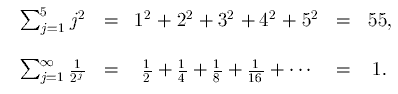but ∑ generally is not used for adding uncountably many things.

(Occasionally it is so used: The sum of an arbitrary collection of nonnegative real numbers is the sup of the sums of finitely many members of that collection. But all the interesting action is happening on a countable set. It can be proved that if more than countably many of those numbers being added are nonzero, the sum must be infinity. Also, there may be other, more esoteric uses for the symbol ∑. But this web page is intended for undergraduates.)

However, in some sense we add together uncountably many things when we use an integral. An equation such as ∫ 3x2dx = x3+C says that we add together uncountably many infinitesimals, and we get a medium-sized number. An equation such as ∫ 3x2 = x3+C couldn't possibly be right -- it says we add together uncountably many medium-sized numbers and get a medium-sized number.

A related difficulty is in trying to understand what "differentials" are. Most recent calculus books have a few pages on this topic, shortly before or after the Chain Rule. I am very sorry that the authors of calculus books have chosen to cover this topic at this point in the book. I think they are making a big mistake in doing so. When I teach calculus, I skip that section, with the intention of covering it in a later semester. Here is why:

When y=f(x), then dy=f′(x)dx is really a function of two variables-- it is a function of both x and dx. But in many calculus textbooks, that fact is not confronted directly; it is swept under the rug and hidden. Several hundred pages later in most calculus textbooks, we are introduced to functions of two variables, and given a decent notation for them -- e.g., we may have z = h(u,v). At this point the student may begin to understand functions of two variables, and we have partial derivatives etc. But before this point, we are not given any good notations for a function of two variables. Our beginning math students have difficulty enough with abstractions even when they are provided with decent notation; how can we expect them to think abstractly without the notation? Thus, when I teach calculus, I describe "dx" and "dy" as "pieces of the notation dy/dx," with no independent meanings of their own. I think that this approach is much kinder to the beginning students.This web page was selected as the "cool math web page of the week", for the week of May 22, 2002, by KaBoL.